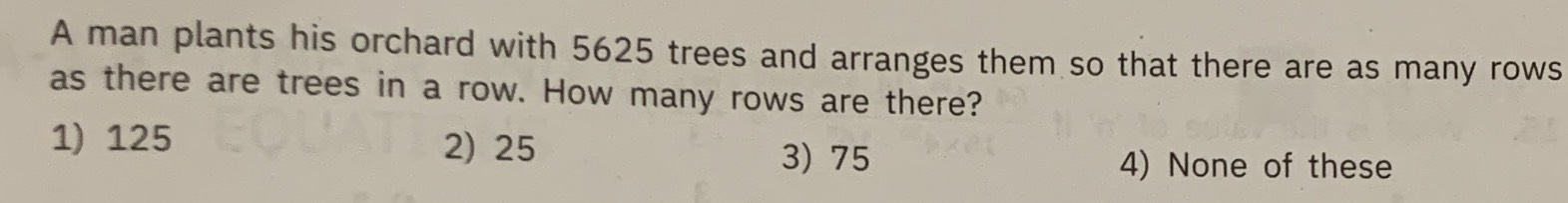### ¿Todavía tienes preguntas de matemáticas?

Pregunte a nuestros tutores expertos
Algebra
PreguntaA man plants his orchard with $$5625$$ trees and arranges them so that there are as many rows as there are trees in a row. How many rows are there?

1) $$125$$ 2) $$25$$ 3) $$75$$ 4) None of these